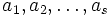# Orthogonal projection formula

## Statement

### Splitting field case using bilinear product

Let$G$ be a finite group and$k$ a field whose characteristic does not divide the order of$G$. Suppose$\varphi:G \to GL(V)$ is a finite-dimensional linear representation of$G$ over$k$. Suppose further that$k$ is a splitting field for$G$.

By Maschke's lemma,$\varphi$ must be completely reducible i.e. it is the direct sum of irreducible representations. Suppose the irreducible representations are$\varphi_1, \varphi_2, \ldots, \varphi_s$ and their multiplicities are$a_1, a_2, \ldots, a_s$ respectively. Then if$\chi_i$ is the character of$\phi_i$ and$\chi$ of$\phi$ we have:$\langle \chi,\chi_i \rangle_G = a_i$

where$\langle \ , \ \rangle_G$ is the Inner product of functions (?):$\langle f_1,f_2 \rangle = \frac{1}{|G|} \sum_{g \in G} f_1(g)f_2(g^{-1})$

Also$\chi$ is orthogonal to the character of any irreducible linear representation not among the$\varphi_i$s, i.e.,$\langle \chi, \alpha \rangle = 0$ for any such$\alpha$.

### Non-splitting field case

Let$G$ be a finite group and$k$ a field whose characteristic does not divide the order of$G$. Suppose$\varphi:G \to GL(V)$ is a finite-dimensional linear representation of$G$ over$k$. Suppose further that$k$ is a splitting field for$G$.

By Maschke's lemma,$\varphi$ must be completely reducible i.e. it is the direct sum of irreducible representations. Suppose the irreducible representations are$\varphi_1, \varphi_2, \ldots, \varphi_s$ and their multiplicities are$a_1, a_2, \ldots, a_s$ respectively. Then if$\chi_i$ is the character of$\phi_i$ and$\chi$ of$\phi$ we have:$\langle \chi,\chi_i \rangle_G = a_id$

where$d$ is a positive integer, which is actually the sum of squares of degrees of irreducible constituents of$\varphi_i$ when it is decomposed over a splitting field.

As before$\langle \ , \ \rangle_G$ is the inner product of functions:$\langle f_1,f_2 \rangle = \frac{1}{|G|} \sum_{g \in G} f_1(g)f_2(g^{-1})$

### Alternate version for characteristic zero using a Hermitian inner product

If$k$ is a subfield of the complex numbers closed under complex conjugation, we can, instead of using the inner product specified here, use the Hermitian inner product (see Inner product of functions#Hermitian inner product):$\langle f_1, f_2 \rangle_G = \frac{1}{|G|} f_1(g)\overline{f_2(g)}$

It so turns out that when restricted to characters of representations, the Hermitian inner product coincides with the inner product used in general. See Inner product of functions#Relation between the definitions.

### Key distinction between characteristic zero and prime characteristic

Although the statement above is valid in characteristic zero and in prime characteristics not dividing the order of the group, there is a key distinction:

• In characteristic zero, the inner product value is an element living in a field of characteristic zero, and so the equality$\langle \chi,\chi_i \rangle_G = a_i$ allows us to retrieve either side from the other.
• In characteristic$p$, the inner product value is in a field of characteristic$p$, whereas the multiplicity$a_i$ is an actual nonnegative integer. So the equality$\langle \chi,\chi_i \rangle_G = a_i$ for splitting fields needs to be interpreted more carefully: given$a_i$, we know$\langle \chi, \chi_i \rangle_G$ uniquely as$a_i \pmod p$. However, the value$\langle \chi,\chi_i \rangle_G = a_i$ only tells us$a_i \pmod p$ and does not allow us to disambiguate between the various possibilities for$a_i$. The same caveat applies to non-splitting fields.

## Facts used

1. Maschke's averaging lemma: This just guarantees complete reducibility.
2. Character orthogonality theorem

## Proof

### Splitting field case

Given:$\varphi$ is a representation of a finite group$G$ over a splitting field$k$, and it reduces completely as a sum of$a_1$ copies of$\varphi_1$,$a_2$ copies of$\varphi_2$, and so on till$a_s$ copies of$\varphi_s$, All the$\varphi_i$ are distinct irreducible representations.$\chi_i$ is the character of$\varphi_i$.

To prove:$\langle \chi, \chi_i \rangle_G = a_i$. Further, if$\psi$ is an irreducible representation not among the$\varphi_i$s, and$\alpha$ is its character, then$\langle \chi, \alpha \rangle_G = 0$.

Step no. Assertion/construction Facts used Given data used Previous steps used Explanation
1$\chi = \sum_{i=1}^s a_i\chi_i$ Trace is linear$\varphi$ is a sum of$\varphi_i$s
2$\langle \chi_i, \chi_i \rangle_G = 1$ and$\langle \chi_i,\chi_j \rangle_G = 0$ for$i \ne j$ Fact (2)$k$ is a splitting field,$\varphi_i$ are irreducible.
3 We get$\langle \chi, \chi_i \rangle = a_i$ by additivity of the inner product Step (2) Combine Step (2) and additivity of the inner product.
4$\langle \chi_i, \alpha \rangle = 0$ for all$i$. Fact (2)$k$ is a splitting field,$\varphi_i$ are irreducible and so is$\psi$.
5$\langle \chi, \alpha \rangle = 0$ Step (4) Combine Step (4) and additivity of the inner product.

## Consequences

### Uniqueness of decomposition as a sum of irreducible representations

The orthogonal projection formula tells us that given a representation, we can determine the multiplicities of irreducible representations in it. Thus, a representation cannot be expressed as a sum of irreducible representations in more than one way.

### Character determines the representation

In characteristic zero, a representation is determined upto equivalence, by its character. This is essentially because the character determines the multiplicities of the irreducible constituents, which in turn determines the representation uniquely. We can rephrase this as: any field of characteristic not dividing the order of a finite group, is a character-determining field for the group. That is, every representation over the field is uniquely determined by its character.

Further information: Character determines representation in characteristic zero

### Regular representation as a sum of irreducible representations

The orthogonal projection formula can be used to show that the regular representation is:$\sum d_i\chi_i$

where$\chi_i$ are the characters of irreducible linear representations, and$d_i$ is the degree of$\chi_i$.